Molecule Types Linear Molecules

# Linear Molecule Hamiltonian

The linear molecule Hamiltonian used by PGOPHER is designed to be absolutely standard. IUPAC has produced a set of recommendations for fine and hyperfine parameters (Hirota et al, 1994) which are followed by PGOPHER as far as possible. The basic form ignoring centrifugal distortion is the sum of the terms in the following terms in the table:
 For all states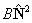Rotation S > 0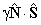Spin-Rotation Λ > 0, S > 0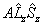Spin-Orbit S > 1/2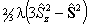Spin-Spin S > 1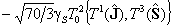Spin-Rotation Λ > 0, S > 1Spin-Orbit S > 3/2Spin-Spin Π statesΛ doubling Π states, S > 0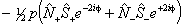Λ doubling Π states, S > 1/2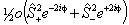Λ doubling Δ states See Λ type doubling for Δ and higher states.

See the individual sections below for a more detailed discussion. Note that any operators not listed above (such as alternate forms of operators or higher powers of centrifugal distortion) can be generated using a perturbation diagonal in electronic state.

## The Rotational Operator

The standard way of writing the rotational kinetic energy is in terms of the angular momentum excluding spin,: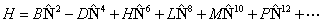though formally, it should only involve the rotational angular momentum of the nuclear framework,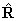and thus be written: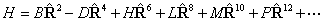PGOPHER can use either form, as controlled by the "RSquaredH" flag at the molecule level. This flag affects many of the terms in the Hamiltonian; for all the linear molecule terms read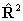for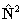if RSquaredH=True; to follow the IUPAC recommendations theform must be used.

The reason both forms are in use is that each has some problems in evaluation, as discussed by Brown et al, 1987. Strictly the second form is correct, but full evaluation is not possible because of the terms involving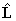. Consider evaluating the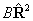term: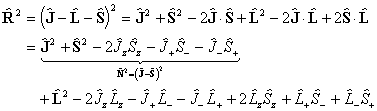The diagonal terms are: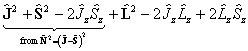which becomes: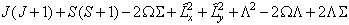The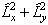term must be discarded, leaving:which is the form used. The equivalent expression inis:i.e. simply an additional Λ2 term compared to. The main practical difference is a shift in the effective band origin of BΛ2 (so they are identical for Σ states), but it is not clear that one is any more correct than the other as both ignore the term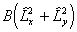. This latter term is only likely to be worth considering if isotope shifts of vibrational band origins are required, and the former leads to different definitions of the band origin, so it is worth checking all published constants to see which is used. The matrix elements of higher powers ofor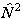are evaluated by evaluating the matrix of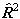oras above and taking the appropriate power of the matrix. This means that the RSquaredH flag will cause small changes which will affect most of the constants so, for example, B will change by 2Λ2D.

Of the off-diagonal terms,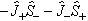is the same in both forms, and their inclusion allows Hund's case (b) to be accurately modelled. The terms off diagonal in Λ: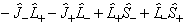are normally discarded in both forms of the Hamiltonian as they only connect completely different electronic states so they are not normally important. They can be included as a perturbation if required (see Luncouple)

## Spin Orbit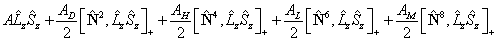where: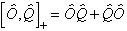Note that, for 2Π states AD and the spin rotation constant γ (see below) have the same effect on the energy levels so their effects can only be distinguished by special methods (Veseth, 1971 and Brown and Watson, 1977). For this reason only one of AD and γ is normally used. A similar equivalence applies in practice for higher order centrifugal distortion terms, so these were only implemented in 10.1.158. Any required centrifugal term can be generated using perturbations if required.

For states with S > 1 an additional term is required:as described in Brown et al, 1981.

## Spin-Spin

For S > 1/2 the spin-spin interaction can contribute: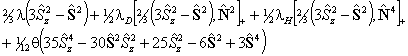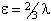is also in use. The term in θ only contributes for states with S > 3/2; again see references in Brown et al, 1987. Read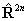for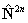if RSquaredH=True.

## Spin-Rotation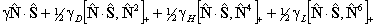If RSquaredH=True, readforbut note theterm is unchanged. For S > 1 an additional term is also required:This form is from Cheung et al, 1984. It is perhaps clearer in terms of its (only) Hund’s case (a) matrix elements as given by Brown and Milton, 1976: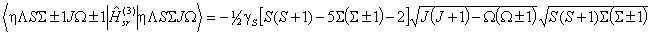## Λ type doubling for Π states

The IUPAC form is: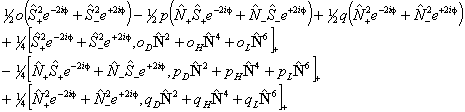Of these terms q will contribute for any Π state; for 1Π states it is effectively the difference between the rotational constants for e and f parity states. p requires S > 0 also and o will only contribute for S > 1/2. The e±2iφ terms are shorthand to ensure that the Λ-doubling operators only connect the two halves of a Π state: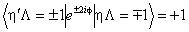If RSquaredH=True, readforin the centrifugal distortion terms, and leave the other operators unchanged. There are alternative Λ doubling parameters, in use including: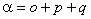This arises naturally from expressing the Hamiltonian in terms of J, rather than N as can be seen by making the replacement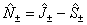: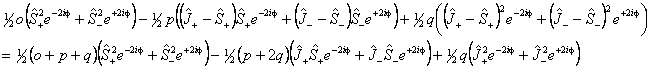See Brown and Merer, 1979 for a discussion of this. This also explains why under some circumstances only p + 2q is determined rather than p and q individually. (A term equivalent to this can be generated using perturbations; see the CrO sample file for an example of this.)

## Λ type doubling for Δ and higher states

Λ doubling in Δ states is discussed by Brown et al, 1987. They propose two different forms, a case (b) form: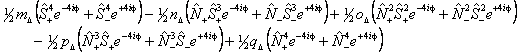and a case (a) form: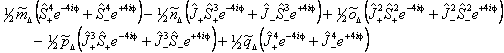The case (a) form is programmed into PGOPHER with the non-standard names for the parameters: m̃Δ = o, ñΔ = oD, õΔ = oH, p̃Δ = p and q̃Δ = q, but does not include any centrifugal distortion terms. The case (b) form, and centrifugal distortion of either can be generated using perturbation objects, and Λ doubling operators for higher values of Λ can also be generated in this way.

## References

• "Spin-dependent interactions for linear molecules in Σ states of quartet and higher multiplicity", J. M. Brown and D. J. Milton, Mol. Phys, 31, 409 (1976).
• "Lambda-type doubling parameters for molecules in Π electronic states of triplet and higher multiplicity", J. M. Brown and A. J. Merer, J. Mol. Spec., 74, 488 (1979).
• "Higher-order fine structure of the a4Πu state of O2+. J. M. Brown, D. J. Milton, J. K. G. Watson, R. N. Zare, D. L. Albritton, M. Horani and J Rostas, J. Mol. Spec., 90, 139 (1981).
• "Λ-type doubling parameters for molecules in Δ electronic states", J. M. Brown, A. S-C. Cheung and A. J. Merer, J. Mol. Spec., 124, 464 (1987).
• "Spin-orbit and spin-rotation coupling in doublet states of diatomic molecules", J. M. Brown and  J. K. G. Watson, J. Mol. Spec., 65, 65 (1977).
• "Fourier transform spectroscopy of CrO: Rotational analysis of the A5Σ – X5Π (0,0) band near 8000 cm–1.", A. S-C. Cheung, W. Zyrnicki and A. J. Merer, J. Mol. Spec., 104, 315 (1984).
• "Symbols for fine and hyperfine parameters", E. Hirota, J. M. Brown, J. T. Hougen, T. Shida and N. Hirota, Pure & Appl. Chem., 66, 571 (1994).
• "Corrections to the spin-orbit splitting in 2Π states of diatomic molecules", L. Veseth, J. Mol. Spec., 38, 228 (1971).